Next: Menger universal curve Up: Cyclic examples of locally Previous: Menger universal continua

## Sierpinski carpet

The Sierpinski universal plane curve or the Sierpinski carpet [Sierpinski 1916]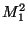is a well known fractal obtained as the set remaining when one begins with the unit square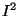and applies the operation of dividing it into 9 congruent squares and deleting the interior of the central one, then repeats this operation on each of the surviving 8 squares, and so on. See Figure A.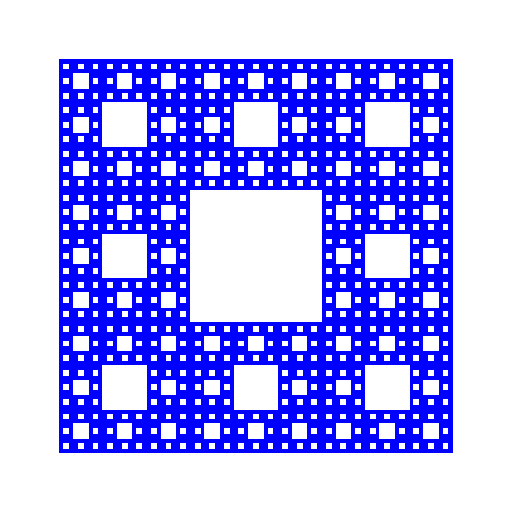1.is universal in the class of all at most one-dimensional subsets of the plane (equivalently, of all boundary subsets of the plane) [Sierpinski 1916], [Sierpinski 1922].
2. The following statements are equivalent:
1.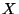is homeomorphic to;
2.is a locally connected plane curve that contains no local cut points;
3.is a continuum embeddable in the plane in such a way that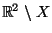has infinitely many components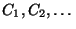such that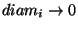,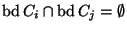for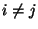,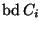is a simple closed curve for each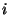and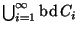is dense in[Whyburn 1958].
3. A complete metric spacecontains a topological copy ofif and only ifcontains a subset with the bypass property [Prajs 1998a].
4. The group of all autohomeomorphisms ofhas exactly two orbits: one of them is the union of all simple closed curves which are the boundaries of complementary domains of[Krasinkiewicz 1969].

The group is a Polish topological group which is totally disconnected and one-dimensional (see [Brechner 1966, Theorem 1.2] and Property 7 in 1.4.1).

5. Any homeomorphism between Cartesian products of copies of the Sierpinski carpet is factor preserving [Kennedy Phelps 1980]. Consequently, no such product is homogeneous.

6. The Sierpinski carpet can be continuously decomposed into pseudo-arcs such that the decomposition space is homeomorphic to the carpet [Prajs 1998b, Corollary 18], [Seaquist 1995]. In fact,is the only planar locally connected curve admitting such a decomposition [Prajs 1998b, Corollary 18].

7. The Sierpinski carpet is homogeneous with respect to monotone open mappings [Prajs 1998b, Corollary 24], [Seaquist 1999]. Moreover, every continuum which is locally homeomorphic to(i. e.,-manifold) is homogeneous with respect to monotone open mappings [Prajs 1998b, Theorem 23].

8.is homogeneous with respect to the class of simple mappings [Charatonik 1984].

9. If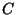is a curve, then the set of all mappings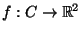such that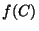is homeomorphic tois a residual subset of the space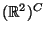of all mappings ofinto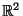with the uniform convergence metric.

If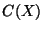is the hyperspace of all subcontinua of a compact spaceand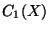its subspace of all curves, then the set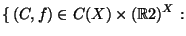$f(C)$ is homeomorphic to $M^2_1$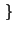is residual in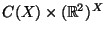; in other words, almost all mappings in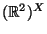map almost all curves inonto copies of, where "almost all" means all with except of a subset of the first category in corresponding spaces [Mazurkiewicz 1938].

10. If a locally compact spacecontains a topological copy of the Sierpinski carpet, then the space of all copies of the Sierpinski carpets inwith the Hausdorff metricHausdorff metric is a true absolute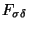-set [Krupski XXXXb].

Here you can find source files of this example.

Here you can check the table of properties of individual continua.

Here you can read Notes or write to Notes ies of individual continua.Next: Menger universal curve Up: Cyclic examples of locally Previous: Menger universal continua
Janusz J. Charatonik, Pawel Krupski and Pavel Pyrih
2001-11-30